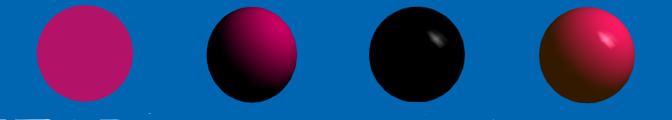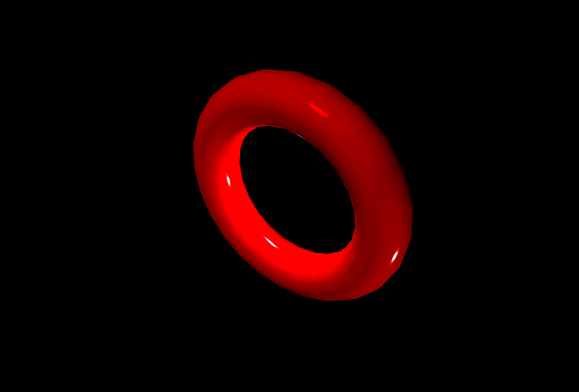# How to Design Phong Shading Graphics using p5.js ?

• Last Updated : 12 Aug, 2021

Phong shading is a specific type of shading technique in 3D computer graphics that is useful for smoothing out multi-surface shapes, approximating surface highlights, and creating more sophisticated computer-modeled images. Experts refer to the technique as “interpolation,” where Phong shading visualizes a smoother surface for a 3D model. The Phong model is also very useful to learn about more advanced rendering techniques.

Phong shading has the following features:

• It is able to produce highlights that occur in the middle of a polygon.
• The lighting computation is performed at every pixel.
• Normal vectors are interpolated over the polygon.

Phong Shading Algorithm: There are three distinct components involved in Phong Shading:

• Ambient: This component approximates light coming from a surface due to all the non-directional ambient light that is in the environment.
• Diffuse: This component approximates light, originally from light source, reflecting from a surface which is diffuse or non glossy.
• Specular: This component determines how much the object shines.

The complete Phong shading model for a single light source is:

`[ra,ga,ba] + [rd,gd,bd]max0(n•L) + [rs,gs,bs]max0(R•L)p`

The model for multiple light sources is:

```[ra,ga,ba] + Σi( [Lr,Lg,Lb]
( [rd,gd,bd]max0(n•Li) + [rs,gs,bs]max0(R•Li)p ) )```

Visual Illustration of Phong Shading: Here the light is white with position x=1, y =1, z=-1. The ambient and diffuse component colors are both purple, the specular color is white which is reflecting a small part of the light hitting the surface of the object in narrow highlights. The intensity of the diffuse component varies with the direction of the surface and due to different light positions. The ambient component is uniformly shaded.AMBIENT + DIFFUSE + SPECULAR = PHONG SHADING

## Javascript

 `function` `setup() { ` `  ``createCanvas(640, 500, WEBGL); ` `} ` ` `  `function` `draw() { ` ` `  `  ``// Setting the vector values  ` `  ``// or the direction of light ` `  ``let dx = 300; ` `  ``let dy = 200; ` `  ``let dz = -600; ` `  ``let v = createVector(dx, dy, dz); ` ` `  `  ``// Creating the ambient light  ` `  ``ambientLight(0, 0,255); ` `   `  `  ``// Creating the directional light ` `  ``// by using the given vector ` `  ``directionalLight(255, 0, 0, v); ` `   `  `  ``shininess(255); ` `  ``specularColor(255); ` `  ``specularMaterial(255); ` `   `  `  ``// Creating the point lights at the ` `  ``// given points from the given directions ` `  ``pointLight(255, 255, 255, 0, -50, 0); ` `  ``pointLight(255, 255, 255, 200,200,30); ` `   `  `  ``rotateX(0.01*frameCount); ` `  ``rotateY(0.01*frameCount); ` `  ``rotateZ(0.03*frameCount); ` `   `  `  ``// Setting the background ` `  ``// to black ` `  ``background(0); ` ` `  `  ``noStroke(); ` ` `  `  ``fill(255, 0, 0); ` `  ``torus(100,25); ` `}`

Output:My Personal Notes arrow_drop_up
Recommended Articles
Page :